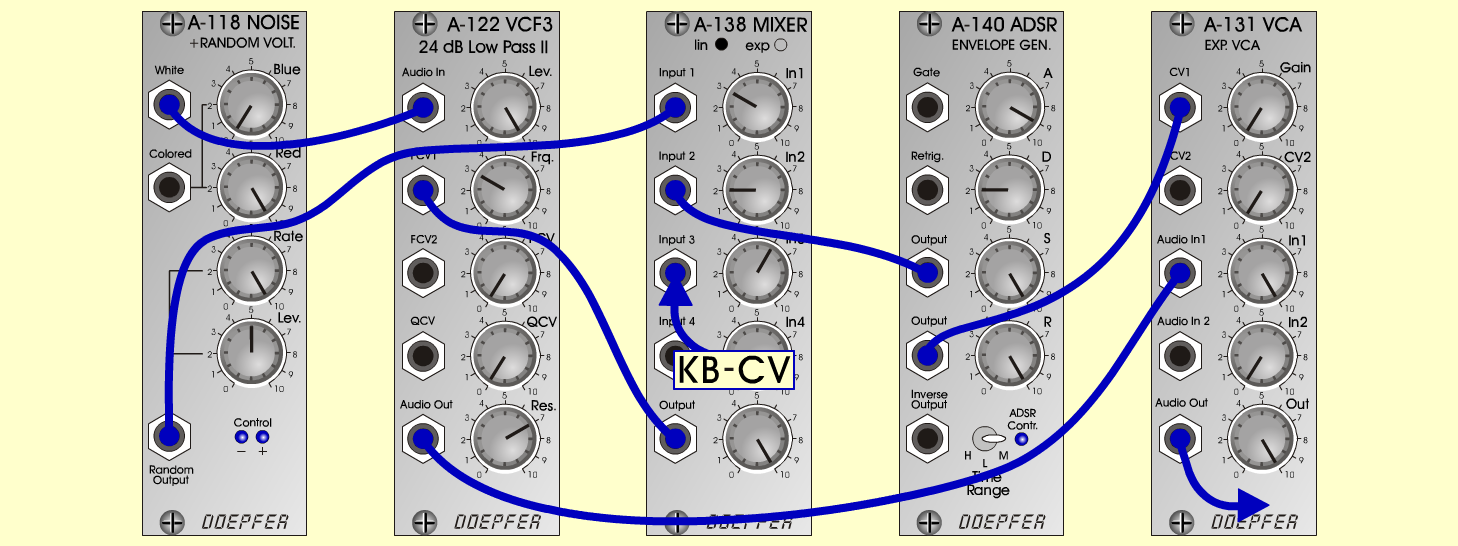StormyN a t u r eD o e p f e r A - 100 Connections: Settings: A-118 (White)  <=>  A-122 (Audio In) A-122 (Audio Out)  <=>  A-131 (Audio In 1) A-118 (Random)  <=>  A-138lin (Input 1) A-140 (Output 1)  <=>  A-138lin (Input 2) KB (CV)  <=>  A-138lin (Input 3) A-138lin (Output)  <=>  A-122 (FCV 1) A-140 (Output 2)  <=>  A-131 (CV 1) KB (Gate)  <=>  A-140 (Gate) A-131 (Audio Out)  <=> Amplifier A-118 (Blue = 0, Red = 10, Rate = 10, Level = 5) A-122 (Level = 10, Freq = 3, Res = 7) A-138 (In 1 = 3, In 2 = 2, In 3 = 6, Out = 10) A-140 (A = 9, D = 2, S = 10, R = 10, Range = M) A-131 (Gain = 0, Audio In 1 = 10,  Audio Out = 10) Josef MuellerSound samples Bad weather (with Rain & Thunder) Stormy